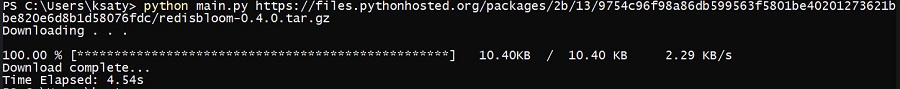Open in App
Not now

• Difficulty Level : Medium
• Last Updated : 24 Jan, 2021

Python is one of the most popular general-purpose programming languages with a wide range of use cases from general coding to complex fields like AI. One of the reasons for such popularity of python as a programming language is the availability of many built-in as well as third-party libraries and packages.

#### Approach:

• Take the file URL via command line
• Open the file input or download stream over HTTP via requests.
• Display the details
• Save the file.

### Step-by-step implementation:

Step 1: Libraries required

## Python3

 `import` `requests``import` `sys``import` `time`

Step 2: a dictionary for detecting file size in proper units

## Python3

 `units ``=` `{``'B'``:{``'size'``:``1``, ``'speed'``:``'B/s'``},``'KB'``:{``'size'``:``1024``, ``'speed'``:``'KB/s'``},``'MB'``:{``'size'``:``1024``*``1024``, ``'speed'``:``'MB/s'``},``'GB'``:{``'size'``:``1024``*``1024``*``1024``, ``'speed'``:``'GB/s'``}``}`

Function for checking units of the length of the file downloaded. Length is in bytes so corresponding units are checked.

## Python3

 `def` `check_unit(length): ``# length in bytes``  ``if` `length < units[``'KB'``][``'size'``]:``    ``return` `'B'``  ``elif` `length >``=` `units[``'KB'``][``'size'``] ``and` `length <``=` `units[``'MB'``][``'size'``]:``    ``return` `'KB'``  ``elif` `length >``=` `units[``'MB'``][``'size'``] ``and` `length <``=` `units[``'GB'``][``'size'``]:``    ``return` `'MB'``  ``elif` `length > units[``'GB'``][``'size'``]:``    ``return` `'GB'`

We will open a file stream over HTTP using requests and then save the data in chunks to the local file. Let’s see how the code will look and then put it all together.

## Python3

 `# Opening file stream`` ` `r ``=` `requests.get(link_to_file, stream ``=` `True``)  `` ` `# writing file data in chunks.``# for examples, A file of 10 MB written in``# chunk size of 8096 Bytes.``with ``open``(file_name, ``'wb'``) as f:  ``  ``for` `chunk ``in` `r.iter_content(chunk_size):  ``    ``f.write(chunk)`

Step 4: Printing output

The output will consist of a dynamic progress bar with downloading details. For this we use stdout.write() and stdout.flush() methods

## Python3

 `sys.stdout.write(format_string ``%` `(list_of_variables))`` ` `# for printing details in single ``# line which updates without going to the next line``# once the details are updated.`` ` `sys.stdout.flush() `

Below is the full implementation:

## Python3

 `import` `requests``import` `sys``import` `time`` ` `units ``=` `{    ``'B'` `: {``'size'``:``1``, ``'speed'``:``'B/s'``},``'KB'` `: {``'size'``:``1024``, ``'speed'``:``'KB/s'``},``'MB'` `: {``'size'``:``1024``*``1024``, ``'speed'``:``'MB/s'``},``'GB'` `: {``'size'``:``1024``*``1024``*``1024``, ``'speed'``:``'GB/s'``}``}`` ` `def` `check_unit(length): ``# length in bytes``    ``if` `length < units[``'KB'``][``'size'``]:``        ``return` `'B'``    ``elif` `length >``=` `units[``'KB'``][``'size'``] ``and` `length <``=` `units[``'MB'``][``'size'``]:``        ``return` `'KB'``    ``elif` `length >``=` `units[``'MB'``][``'size'``] ``and` `length <``=` `units[``'GB'``][``'size'``]:``        ``return` `'MB'``    ``elif` `length > units[``'GB'``][``'size'``]:``        ``return` `'GB'`` ` `# takes download link and directory where file to be saved.``def` `downloadFile(url, directory) :`` ` `    ``localFilename ``=` `url.split(``'/'``)[``-``1``] ``# files name`` ` `    ``with ``open``(directory ``+` `'/'` `+` `localFilename, ``'wb'``) as f:``        ``print` `(``"Downloading . . .\n"``)``        ``start ``=` `time.time() ``# start time``        ``r ``=` `requests.get(url, stream``=``True``)`` ` `        ``# total length in bytes of the file``        ``total_length ``=` `float``(r.headers.get(``'content-length'``)) `` ` `        ``d ``=` `0` `# counter for amount downloaded `` ` `        ``# when file is not available``        ``if` `total_length ``is` `None``:``            ``f.write(r.content)``        ``else``:``            ``for` `chunk ``in` `r.iter_content(``8192``):``                 ` `                ``d ``+``=` `float``(``len``(chunk))``                ``f.write(chunk) ``# writing the file in chunks of 8192 bytes`` ` `                ``# amount downloaded in proper units``                ``downloaded ``=` `d``/``units[check_unit(d)][``'size'``]``                 ` `                ``# converting the unit of total length or size of file from bytes.``                ``tl ``=` `total_length ``/` `units[check_unit(total_length)][``'size'``] ``                 ` `                ``trs ``=` `d ``/``/` `(time.time() ``-` `start) ``# speed in bytes per sec``                 ` `                ``#speed in proper unit``                ``download_speed ``=` `trs``/``units[check_unit(trs)][``'size'``]``                 ` `                ``speed_unit ``=` `units[check_unit(trs)][``'speed'``] ``# speed in proper units`` ` `                ``done ``=` `100` `*` `d ``/` `total_length ``# percentage downloaded or done.``                 ` `                ``fmt_string ``=` `"\r%6.2f %s [%s%s] %7.2f%s  /  %4.2f %s  %7.2f %s"``                 ` `                ``set_of_vars ``=` `( ``float``(done), ``'%'``,``                                ``'*'` `*` `int``(done``/``2``),  ``                                ``'_'` `*` `int``(``50``-``done``/``2``),  ``                                ``downloaded, check_unit(d),  ``                                ``tl, check_unit(total_length),  ``                                ``download_speed, speed_unit)`` ` `                ``sys.stdout.write(fmt_string ``%` `set_of_vars)`` ` `                ``sys.stdout.flush()`` ` `    ``return` `(time.time() ``-` `start) ``# total time taken for download`` ` `def` `main() :``    ``directory ``=` `'.'``    ``if` `len``(sys.argv) > ``1` `:``        ``url ``=` `sys.argv[``1``] ``# url from cmd line arg``        ``if` `len``(sys.argv) > ``2``:``            ``directory ``=` `sys.argv[``2``]``         ` `        ``total_time ``=` `downloadFile(url, directory)``        ``print` `('')``        ``print` `(``"Download complete..."``)``        ``print` `(``"\rTime Elapsed: %.2fs"` `%` `total_time)``    ``else` `:``        ``print``(``"No link found!"``)`` ` `if` `__name__ ``=``=` `"__main__"` `:``    ``main()`

Save the code in a python file and use it as follows

`python <program_name>.py <file_link> <save_location(by default '.')>`

Output:My Personal Notes arrow_drop_up